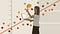# PART 1 : Assumptions of Simple Linear Regression Model and Estimation of Unknown Parameters by Using the Method of Least-SquaresSource

# ☆╮What is the purpose of regression analysis?

Regression analysis is a set of statistical methods used for describing the relationships between independent variables and dependent variable. Our main goal is to estimate the relationships between dependent variable(also known as response variable) y and one or more independent (also known as predictor or explanatory) variables (X). It can be used to evaluate the strength of the relationship between variables for modeling the future relationship between them.

# ╰☆╮Simple Linear Regression Model## AYŞE DUMAN

I am an undergraduate student at Yıldız Technical University Department of Mathematical Engineering and Statistics.